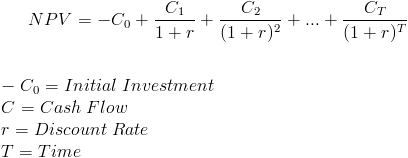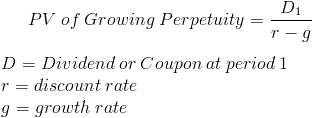expert
Experte mit der besten Antwort

Alessandro

100% Empfehlungsrate

66 Meetings

149 USD / Coaching

# Net present value calculation for both perpetuity and for fix period

Hi,

What are the formulas for npv for perpetuity and fixed period? I am seeing all sorts of formulas online and cannot tell which is what I need

2 Antworten

• Datum aufsteigend
• Datum absteigend

Hey there,

If you don't have an MBA, the likelihood of having to use the formula in an interview (at least for MBB) is close to zero. If you did, you'd probably just to have to discount a couple cash flows in the future (i.e. a manual DCF) rather than just calculating the NPV using the NPV formula. If you are applying to different firms, you should check, but again I would be surprised.

Anyways, to answer your question - for a fix period you would just discount each cash flow year by year, see below:In perpetuity, you just divide the cash flow by the (discount rate - the growth in the cash flow):Note that if there is no growth in cash flow, then g=0, and the formula is D/r.

Hi,

Check this presentation:

I believe you need to understand the concept rather than just apply the formulas. So google can help you here.

Best

## Verwandte BootCamp-Artikel

### Net Present Value - NPV

Use the Net Present Value (NPV) to compare investments with different volatile cash-flows over time and quantitatively assess their attractiveness.

### Valuation

Valuation case studies require you to estimate how much a firm, patent, or service is worth. For these cases, use the Discounted Cash Flow method or the Industry multiple method.